# Some suggestions about Python SDK

• hello，this is my suggestions about Python SDK. Although I am just a primary user ,it maybe useful to develop sdk.

1.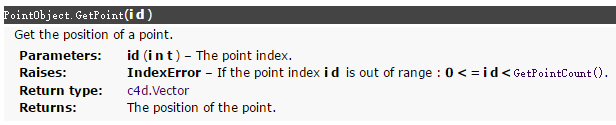GetPoint() isn't return accurate postion, just initial postion.please note or append another method(maybe already have?)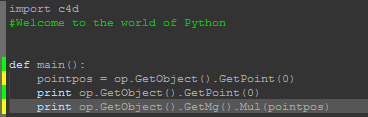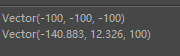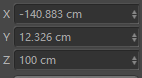this my test , use python tag, box(polygon) and point 0

1. Matrix.mul(other) (matrix * matrix)
ususlly computational order is 'left to right' , such as handwriting or Matlab.but here is 'right to left' ,could you make some changes?it is trouble to adapt.

3.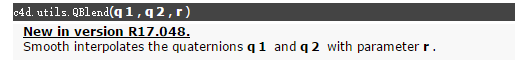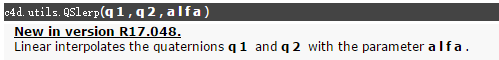maybe c4d.utils.QSlerp(q1,q2,alfa) is 'spherical interpolation' and c4d.utils.QBlend(q1, q2, r) is 'Linear interpolates '?

• sorry my bad typesetting• Hi mike, first of all thanks for providing feedback on the Python API.

1. GetPoint() isn't return accurate postion, just initial postion.please note or append another method(maybe already have?)

PointObject::GetPoint() is indeed accurate and it's supposed to return the position of a point regardless of the affine transformation operated by the global transformation matrix belonging to the BaseObject the PointObject inherits from. This grant a proper design where linear motion-blur doens't require the points to effectively move in space.

1. Matrix.mul(other) (matrix * matrix)
ususlly computational order is 'left to right' , such as handwriting or Matlab, but here is 'right to left' ,could you make some changes?it is trouble to adapt.

Matrix::Mul() actually is used to left multiply a vector by a matrix

``````    transformed_vector = transformation_matrix.mul(original_vector)
``````

To multiply matrices instead simply use the "*"operator

``````    matA = c4d.Matrix()
matA.v1 = c4d.Vector(3.0, 9.0, 27.0)
matB = c4d.Matrix()
matB.v3 = c4d.Vector(8.0, 4.0, 2.0)
print matA * matB
print matB * matA
``````

3.maybe c4d.utils.QSlerp(q1,q2,alfa) is 'spherical interpolation' and c4d.utils.QBlend(q1, q2, r) is 'Linear interpolates '?

Yes, your guess it's right. c4d.utils.QSlerp is spherical interpolation for quaternions whilst c4d.utils.QBlend is the linear interpolation.

Last but not least, please mark your initial post as "Ask as a question" from the Topic Tools menù, and if the answer provide is correct don't forget to set the answering post as "Mark this post as correct answer" from the three-vertical dots menu.

Best, Riccardo

• @r_gigante Thank you for your answer!where is "Mark this post as correct answer" ? There are only two options.

• Hi Mike, please check this thread to properly use the Q&A functionality.

Cheers, Riccardo